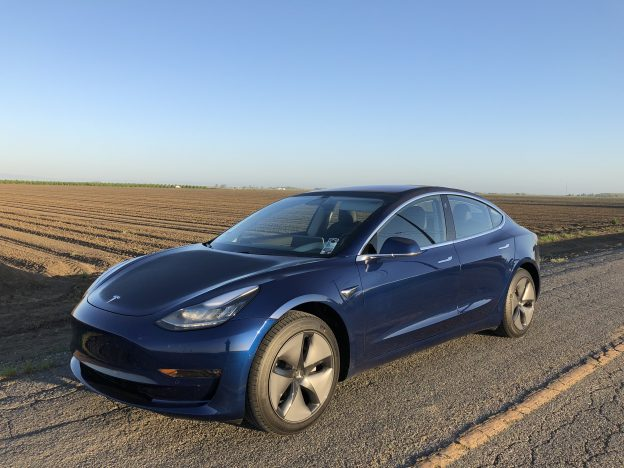# MPGs and Electric Cars

One way to compare gasoline powered cars to one another is fuel economy, the miles per gallon rating that the EPA gives to each car. The average car in the United States gets about 25 MPG. So how can we compare the efficiency of electric vehicles (EVs) with gas-powered cars since EVs store electricity in the battery pack?

The answer is to consider how much energy is stored in a gallon of gas, and how much energy is stored in the battery pack of an EV. The energy in electricity is measured in Watt hours (Wh) or kiloWatt hours (kWh). One kWh = 1000 Wh. An EV might have a battery pack that stores 40, 60, or 100 kWh of energy.

A gallon of gas contains 33.7 kWh of energy. While the average gas powered car in the US travels 25 miles on a gallon of gas. Most EVs will travel over 100 miles on the equivalent amount of energy because electric motors are more efficient.

So let’s consider a new electric car that is rated at 126 MPGe by the EPA. That 126 MPGe rating means the car will travel 126 miles on 33.7 kWh of electricity, which is again the amount of energy stored in one gallon of gas.

Now let’s consider a trip where you drive 300 miles and use 75 kWh of electricity. To calculate the efficiency (75 kWh ÷ 33.7 kWh/gallon = 2.225 gallons of gas, equivalent) we see that 75 kWh equals the equivalent of 2.225 gallons of gas. So 300 miles ÷ 2.225 gallons = 134 MPGe for that hypothetical trip.

The increased efficiency of electric motors is one of the reasons why EVs greatly reduce pollution and emissions. The source of the energy powering an EV is another reason. In regions where electric generation includes hydro, wind, solar, or geothermal the amount of pollution and emissions from driving is further reduced.